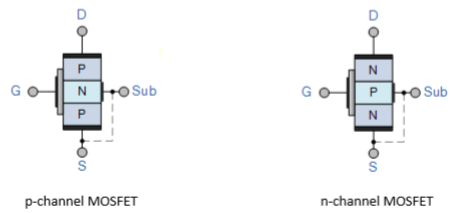# Power Electronics - MOSFET

Metal Oxide Semiconductor Field Effect Transistor (MOSFET) is a type of transistor used to switch electronic signals. It has four terminals namely; source (S), Drain (D), Gate (G) and Body (B).The MOSFET’s body is normally connected to the terminal of the source(S), which results in three-terminal device similar to other field effect transistors (FET). Since these two main terminals are usually interconnected via short circuit, only three terminals are visible in electrical diagrams.

It is the most common device in circuits that are both digital and analogue. Compared to the regular transistor, a MOSFET needs low current (less than one mill-ampere) to switch ON. At the same time, it delivers a high current load of more than 50 Amperes.

## Operation of a MOSFET

MOSFET has a thin layer of silicon dioxide, which acts as the plate of a capacitor. The isolation of the controlling gate raises the resistance of the MOSFET to extremely high levels (almost infinite).

The gate terminal is barred from the primary current pathway; thus, no current leaks into the gate.

MOSFETs exist in two main forms −

• Depletion state − This requires the gate-source voltage (VGB) to switch the component OFF. When the gate is at zero (VGB) the device is usually ON, therefore, it functions as a load resistor for given logic circuits. For loading devices with N-type depletion, 3V is the threshold voltage where the device is switched OFF by switching the gate at negative 3V.

• Enhancement state − The gate-source voltage (VGB) is required in this state to switch the component ON. When the gate is at zero (VGB) the device is usually OFF and can be switched ON by ensuring the gate voltage is higher than the source voltage.

## Symbol and Basic ConstructionWhere, D − Drain; G − Gate; S − Source; and Sub − Substrate